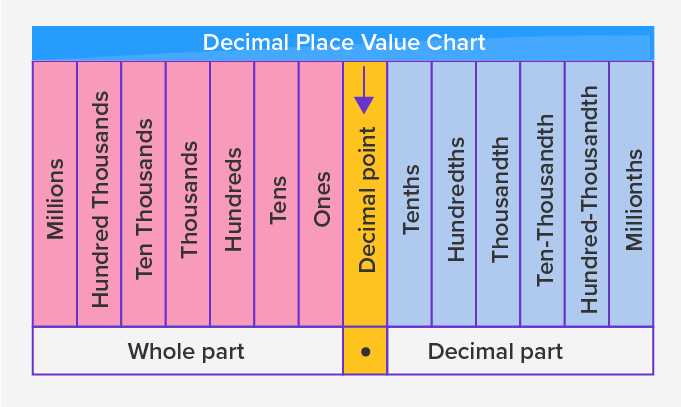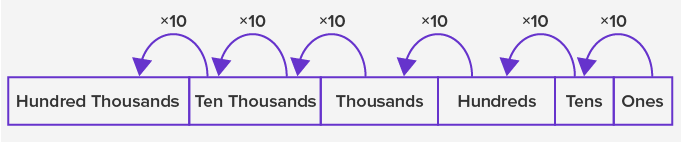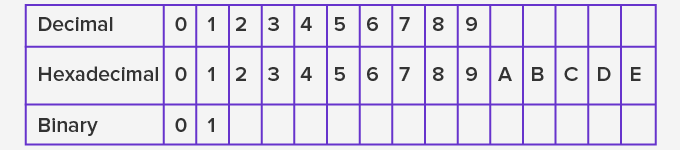Number System - Definition with Examples

The Complete K-5 Math Learning Program Built for Your Child

• 30 Million Kids

Loved by kids and parent worldwide

• 50,000 Schools

Trusted by teachers across schools

• Comprehensive Curriculum

Aligned to Common Core

Number System

Decimal Number System:

The decimal number system consists of 10 digits 0, 1, 2, 3, 4, 5, 6, 7, 8, and 9 and is the most commonly used number system. We use the combination of these 10 digits to form all other numbers. The value of a digit in a number depends upon its position in the number. The place value table for the decimal number system is as:Each place to the left is ten times greater than the place to its right, that is, as we move from the right to the left, the place value increases ten times with each place.• A decimal number system is also called the Base 10 system.

• A number 49,365 is read as Forty-nine thousand three hundred sixty-five, where the value of 4 is forty thousand, 9 is nine thousand, 3 is three hundred, 6 is sixty and 5 is five.

Binary Number System

In the binary number system, we only use two digits 0 and 1. It means a 2 number system.

Example of binary numbers: 1011; 101010; 1101101Each digit in a binary number is called a bit. So, a binary number 101 has 3 bits. 499787080

Computers and other digital devices use the binary system. The binary number system uses Base 2.

The word hexadecimal comes from Hexa meaning 6, and decimal meaning 10. So, in a hexadecimal number system, there are 16 digits. It consists of digits 0 to 9 and then has first 5 letters of the alphabet as:The table below shows numbers 1 to 20 using decimal, binary and hexadecimal numbers.

 Decimal Binary Hexadecimal 0 0 0 1 1 1 2 10 2 3 11 3 4 100 4 5 101 5 6 110 6 7 111 7 8 1000 8 9 1001 9 10 1010 10 11 1011 A 12 1100 B 13 1101 C 14 1110 D 15 1111 E 16 10000 F 17 10001 11 18 10010 12 19 10011 13 20 10100 14

 Fun Facts The decimal number system is also called the the Hindu–Arabic numeral system. Anthropologists hypothesize that the decimal system was the most commonly used number system, due to humans having five fingers on each hand, and ten in both.

Won Numerous Awards & Honors